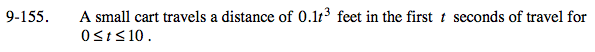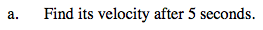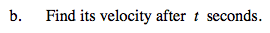### Home > PC > Chapter 9 > Lesson 9.3.5 > Problem9-155

9-155.
1. A small cart travels a distance of 0.1t3 feet in the first t seconds of travel for 0 ≤ t ≤ 10. Homework Help ✎

1. Find its velocity after 5 seconds.

2. Find its velocity after t seconds.First find the slope function.

$\lim_{ h \to 0} \frac{f(t+h)-f(t)}{t+h-t}$

Then find d′(5).

$7.5 \frac{\text{ft}}{\text{sec}}$This is the slope function found above.